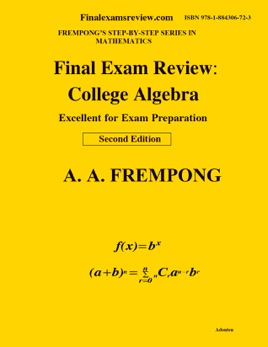• \$18.99

## Publisher Description

Final Exam Review: College Algebra covers the following topics: a note to the student in preparing for exams; Polynomial, Nonlinear, and Radical Equations; Sets, Relations, Functions; Absolute Value Equations and Inequalities; Linear Programming; Graphs of Functions; Asymptotes; Logarithms; Exponential and Logarithmic Equations; Graphs of Exponential and Logarithmic Functions; Matrix and Matrix Methods; Determinants; Complex Numbers and Operations; Polar Form of Complex Numbers; Roots of Complex Numbers; Graphing Polar Coordinates and Equations; Conic sections;; Remainder and Factor Theorems; Rational Roots; Partial Fractions; Sequences and Series; Binomial Theorem; Permutations and Combinations; and Mathematical Induction;